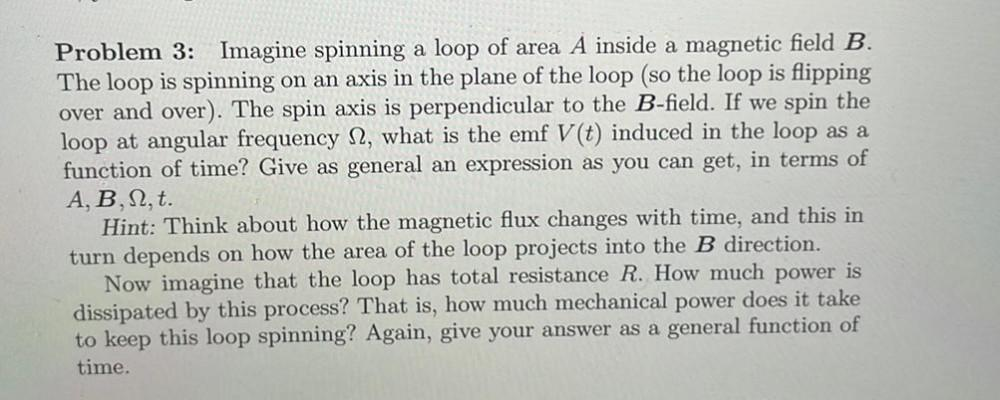Question:

# Problem 3: Imagine spinning a loop of area A inside a magnetic field B. The loop is spinning on an axis in the plane of the loopProblem 3: Imagine spinning a loop of area A inside a magnetic field B. The loop is spinning on an axis in the plane of the loop (so the loop is flipping over and over). The spin axis is perpendicular to the B-field. If we spin the loop at angular frequency 12, what is the emf V(t) induced in the loop as a function of time? Give as general an expression as you can get, in terms of A, B,12, t. Hint: Think about how the magnetic flux changes with time, and this in turn depends on how the area of the loop projects into the B direction. Now imagine that the loop has total resistance R. How much power is dissipated by this process? That is, how much mechanical power does it take to keep this loop spinning? Again, give your answer as a general function of time.